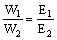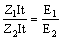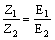# Chemistry Assignment Help with Laws of Electrolysis

## 9.10 Laws of Electrolysis

The laws which govern the deposition of substances (in the form of ions) on electrodes with passage of electric current are called Faraday's law of electrolysis.

The mass of an ion discharged during electrolysis is proportional to the quantity of electricity passed. mathematically,

W µ Q

where, W = Mass of ions liberated in gm, Q = Quantity of electricity passed in coulombs.

But we know that Q = current amperes (I) × Time in sec (t)

\ W µ I × t or W = Z × I × t

In case current efficiency (h) is given, thenW µ Z

Thus electrochemical equivalent may be defined as the mass of the ion deposited by passing a current of one ampere for one second (i.e. by passing coulomb of electricity). Its unit is gram per coulomb.

When the same quantity of electricity is passed through different electrolytes, the masses of different ions liberated at the electrodes are directly proportional to their chemical equivalents (equivalent weights). Suppose W1 and W2 are the weights of the elements deposited by passing a certain quantity of electricity through their salt solutions and E1 and E2 are their respective equivalent weights, then\Thus the electrochemical equivalent (Z) of an element is directly proportional to its equivalent weight (E), i.e.,

E µ Z or E = FZ

Where F is again a proportionality constant and has been found to be 96540 coulombs. It is called faraday.

Thus E = 96540 × Z

1 Faraday = 96500 × coulombs.

Remember that c(coul or C) is the smallest unit of electricity. It is the quantity of electricity passed through a conductor/electrolyte when a current of 1 ampere strength flows for 1 second.,

Coulomb = Amperes × Second since 96500 coulombs = 6.023 × 1023 electrons### Email Based Assignment Help in Laws of Electrolysis

We are the leading online Assignment Help provider. Find answers to all of your doubts regarding the Laws of Electrolysis in chemistry. We at assignmenthelp.net provide homework, Assignment Help to the school, college or university level students. Our expert online tutors are available to help you in Laws of Electrolysis. Our service is focused on: time delivery, superior quality, creativity and originality.